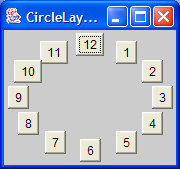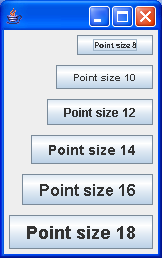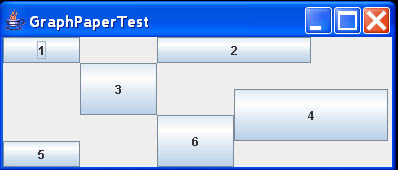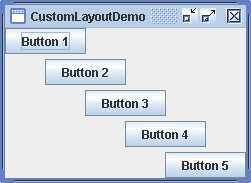# Applet GUI demo of TreeLayout layout manager : Customized Layout « Swing JFC « Java

Applet GUI demo of TreeLayout layout manager

```
import java.applet.Applet;
import java.awt.Button;
import java.awt.Canvas;
import java.awt.Color;
import java.awt.Component;
import java.awt.Container;
import java.awt.Dimension;
import java.awt.Graphics;
import java.awt.Image;
import java.awt.Insets;
import java.awt.Label;
import java.awt.LayoutManager;
import java.awt.List;
import java.awt.Rectangle;
import java.awt.TextField;
import java.util.Enumeration;
import java.util.Hashtable;
import java.util.Vector;

/**
* Applet GUI demo of Gamelan TreeLayout layout manager. Constructs a tree and,
* for each entry, makes a button that jumps to it.
*
* The input language is a file like this: R Java Resources # root L - Resources
* at Sun # label B http://www.sun.com/foo/bar Interesting Stuff # URLbutton B
* http://javasoft.com/b/c More Stuff # URLbutton
*
* The result is (supposedly) a beautiful(?) tree. Each L is a top-level label,
* and each B is in the tree below it.
*
* Could be made much fancier with getParameter("FontName"), "FontSize",
*/
public class TreeLinkTest extends Applet {
TreeLayout tl;

public void init() {
tl = new TreeLayout();
setLayout(tl);
Button root = new Button("This is the root");
tl.setRoot(root);
Component x = new Label("A random label");
tl.setParent(x, root);
Component y;
y = new TextField("Add any component");
tl.setParent(y, root);
x = new List();
tl.setParent(x, root);
x = new Button("Extremely long and unnecessary button title");
tl.setParent(x, y);
x = new MyCanvas(getImage(getDocumentBase(), "icons/tools.gif"));
tl.setParent(x, y);
}

public void paint(Graphics g) {
super.paint(g);
tl.paintLines(g, getBackground());
}

class MyCanvas extends Canvas {
Image img;

MyCanvas(Image img) {
this.img = img;
}

public Dimension getPreferredSize() {
return new Dimension(64, 64);
}

public void update(Graphics g) {
paint(g);
}

public void paint(Graphics g) {
g.drawImage(img, 0, getSize().height / 2 - 16, 32, 32, this);
}
}
}

/**
* Simple layout manager that arranges its children in a tree. The tree always
* expands to fill the available area, and the internal components are resized
* to fit the area proportional to their preferred size and the actual available
* size.
*
* This layout manager requires several method calls beyond the normal layout
* manager. Please notice the following: * Components must be added using the
* add(String, Component) method. The strings don't have to be unique, but must
* be present. * Each instance must have exactly one root object, which must be
* add()ed, <I>then </I> setRoot()ed. * Each component after the root must first
* be added and then must be connected into the tree using setParent(child,
* parent). * If you want lines between parents and children, you <EM>must
* </EM> call paintLines() from your applet's paint() method.
*
* @author name unknown, xxx@blackdown.org
*/

class TreeLayout implements LayoutManager {
TreeNode root;

Hashtable nodes;

public TreeLayout() {
nodes = new Hashtable();
}

public void addLayoutComponent(String name, Component comp) {
TreeNode tn = new TreeNode(comp);
nodes.put(comp, tn);
}

public void removeLayoutComponent(Component comp) {
nodes.remove(comp);
}

/**
* You <em>must</em> make this call, otherwise none of the components will
* be layed out.
*/
public void setRoot(Component c) {
root = (TreeNode) nodes.get(c);
}

/**
* Sets the tree parent of a child. The components <em>must</em> have been
* previously added. If either component has not previously been added, this
* injunction is silently ignored. Cycles are <em>not</em> checked.
*/
public void setParent(Component child, Component parent) {
TreeNode p = (TreeNode) nodes.get(parent);
TreeNode c = (TreeNode) nodes.get(child);
if ((p != null) && (c != null))
}

public Dimension minimumLayoutSize(Container target) {
Dimension d = root.getMinimumSize();
Insets insets = target.getInsets();
d.width += insets.left + insets.right;
d.height += insets.top + insets.bottom;
return d;
}

public Dimension preferredLayoutSize(Container target) {
Dimension d = root.getPreferredSize();
Insets insets = target.getInsets();
d.width += insets.left + insets.right;
d.height += insets.top + insets.bottom;
return d;
}

public void layoutContainer(Container target) {
Insets insets = target.getInsets();
Dimension d = target.getSize();
Dimension root_pref = root.getPreferredSize();
double xscale = ((double) (d.width - insets.left - insets.right) / (double) (root_pref.width));
double yscale = ((double) (d.height - insets.top - insets.bottom) / (double) (root_pref.height));
root.doLayout(xscale, yscale, insets.left, insets.top);
}

/**
* This piece of hackery is needed since one cant really draw things from a
* layout manager. Call this if you want to draw lines between components.
*/
public void paintLines(Graphics g, Color bg) {
Color dk = bg.darker();
Color br = bg.brighter();
root.paintLines(g, dk, br);
}
}

class TreeNode {
Component comp;

Vector children;

Dimension prefSz, minSz;

TreeNode(Component comp) {
super();
this.comp = comp;
children = new Vector();
}

Dimension getMinimumSize() {
if (!comp.isVisible())
return new Dimension(0, 0);
if (minSz == null) {
Dimension d = comp.getMinimumSize();
minSz = new Dimension(d.width, d.height);

if (children.size() > 0) {
for (Enumeration e = children.elements(); e.hasMoreElements();) {
TreeNode t = (TreeNode) (e.nextElement());
if (t.comp.isVisible()) {
d = t.getMinimumSize();
minSz.height += d.height;
minSz.width = Math.max(d.width, minSz.width);
}
}
}
}
return minSz;
}

Dimension getPreferredSize() {
if (!comp.isVisible())
return new Dimension(0, 0);
if (prefSz == null) {
Dimension d = comp.getPreferredSize();
prefSz = new Dimension(d.width, d.height);

if (children.size() > 0) {
int wmax = 0;
for (Enumeration e = children.elements(); e.hasMoreElements();) {
TreeNode t = (TreeNode) (e.nextElement());
if (t.comp.isVisible()) {
d = t.getPreferredSize();
prefSz.height += d.height;
if (wmax < d.width) {
wmax = d.width;
}
}
}
prefSz.width += wmax;
}
}
return prefSz;
}

prefSz = null;
minSz = null;
}

void removeChild(TreeNode t) {
children.removeElement(t);
prefSz = null;
minSz = null;
}

void paintLines(Graphics g, Color dk, Color br) {
if (comp.isVisible()) {
Rectangle b = comp.getBounds();
int x1off = b.x + b.width / 2;
int x2off = b.x + b.width;
int y1off = b.y;
for (Enumeration e = children.elements(); e.hasMoreElements();) {
TreeNode tn = (TreeNode) (e.nextElement());
if (tn.comp.isVisible()) {
Rectangle bn = tn.comp.getBounds();
int y2off = bn.y + bn.height / 2;
g.setColor(dk);
g.drawLine(x1off, y1off, x1off, y2off);
g.drawLine(x1off, y2off - 1, x2off, y2off - 1);
g.setColor(br);
g.drawLine(x1off + 1, y1off, x1off + 1, y2off);
g.drawLine(x1off, y2off, x2off, y2off);
tn.paintLines(g, dk, br);
}
}
}
}

void doLayout(double xscale, double yscale, int x, int y) {
// x and y are the offsets into the
// Container where we start doing the
// goodies for this Node
if (comp.isVisible()) {
Dimension pref = comp.getPreferredSize();
int ht = (int) Math.round(yscale * pref.height);
int wd = (int) Math.round(xscale * pref.width);

ht = (pref.height < ht) ? pref.height : ht;
wd = (pref.width < wd) ? pref.width : wd;

comp.setBounds(x, y, wd, ht);
y += ht;
x += wd;

for (Enumeration e = children.elements(); e.hasMoreElements();) {
TreeNode tn = (TreeNode) (e.nextElement());
if (tn.comp.isVisible()) {
pref = tn.getPreferredSize();
tn.doLayout(xscale, yscale, x, y);
y += (int) Math.round(yscale * pref.height);

}
}
}
}
}

```

### Related examples in the same category

 1 Custom layout: EdgeLayout 2 Customized layout manager3 ColumnLayout4 Relative Layout Manager for Java J2SE 5 Basically two (or more) columns of different, but constant, widths 6 GraphPaperLayout7 Table Layout 8 Table Layout implements LayoutManager2 9 Table layout manager 10 Flex Layout 11 Square Layout 12 Center Layout 13 Wrapper Layout 14 Tile Layout 15 Custom Layout Demo16 X Y Layout 17 DividerLayout is layout that divides two components with the column of actions 18 Stack Layout, uses an orientation to determine if the contents should be arranged horizontally or vertically. 19 A simple layoutmanager to overlay all components of a parent. 20 A layout manager that displays a single component in the center of its container. 21 A layout manager that spaces components over six columns in seven different formats. 22 Compents are laid out in a circle. 23 Special simple layout used in TabbedContainer 24 Place components at exact locations (x, y, width, height) and then determine how they behave when the window containing them (their parent) is resized 25 Specialised layout manager for a grid of components.